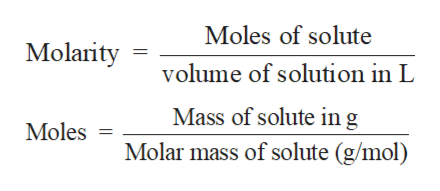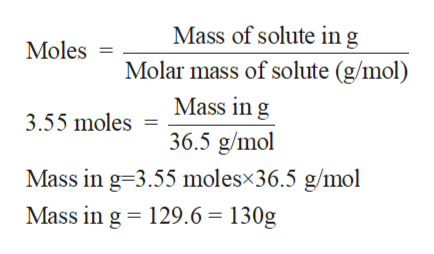Question
15 views

How many grams are in 3.55 moles of NaCl?

check_circle

Step 1

Solution stoichiometry involves the calculation of concentration of solutions in the given conditions of volumes, moles etc.

Step 2

Solution stoichiometry is mainly based on the calculation of moles and volumes. These two values are used to calculate the molarity of solution. The relation between moles, volume and molarity is as given below;help_outlineImage TranscriptioncloseMoles of solute Molarity volume of solution in L Mass of solute ing Molar mass of solute (g/mol) Moles fullscreen
Step 3

Molar mass of HCl = 36.5 g/mol

Moles of HCl = 3.5...help_outlineImage TranscriptioncloseMass of solute ing Molar mass of solute (g/mol) Mass in g Moles 3.55 moles = 36.5 g/mol Mass in g-3.55 molesx36.5 g/mol Mass in g 129.6 130g fullscreen

### Want to see the full answer?

See Solution

#### Want to see this answer and more?

Solutions are written by subject experts who are available 24/7. Questions are typically answered within 1 hour.*

See Solution
*Response times may vary by subject and question.
Tagged in

### Other